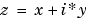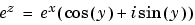MATLAB Function Referenceexp

Exponential

Syntax

• ```Y = exp(X)
```

Description

The `exp` function is an elementary function that operates element-wise on arrays. Its domain includes complex numbers.

```Y = exp(X) ``` returns the exponential for each element of `X`. For complex, it returns the complex exponential.

Remark

Use `expm` for matrix exponentials.

See Also

`expm`, `log`, `log10`, `expint`

© 1994-2005 The MathWorks, Inc.